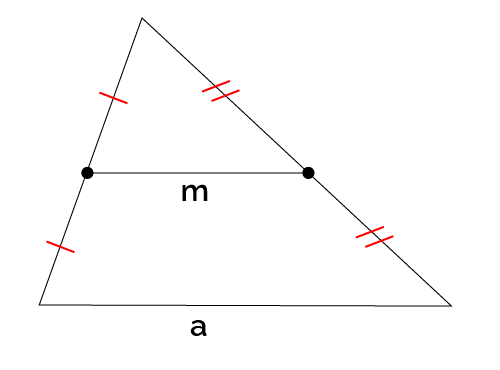# Midsegment of a Triangle Calculator

Created by Anna Szczepanek, PhD
Last updated: Jun 22, 2022

Welcome to Omni's midsegment of a triangle calculator! No matter if you are:

• Not sure what a midsegment of a triangle is;
• Wondering how to find the midsegment of a triangle using a compass and straightedge; or
• Struggling to understand what the midsegment theorem is all about.

With this midsegment of a triangle calculator, you'll quickly become a midsegment expert! Let's start our journey with the definition of a midsegment of a triangle, shall we?

## What is a midsegment of a triangle?

In a triangle, the midsegment is a line that connects the midpoints of two sides of this triangle. And the midpoint of a side is the point that is equidistant from either vertex. Since a triangle has three sides and we can connect the midpoints of any two sides, each triangle has three midsegments.

#### Midsegment theorem

The most important property of midsegments is the following: a midsegment is parallel to the side we did not use to draw this midsegment (called the base) and its length is equal to one-half of the length of this base. This result is known under the name of triangle midsegment theorem.

## How to find the midsegment of a triangle?

It follows directly from the definition of a midsegment of a triangle that in order to find the midsegment, you need to connect the midpoints of two sides. To find the midpoints with a compass:

1. Put the needle on a vertex and draw an arc with a radius greater than half the side.
2. Repeat for the other vertex. Make sure the arcs have two intersection points.
3. Connect the intersection points. The point where you intersect the side is exactly the midpoint of this side.

## How to use this midsegment of a triangle calculator?

Here's a quick guide on how to use this tool efficiently:

1. Choose the mode. Our midsegment of a triangle calculator can solve two types of problems:
• Find the length of the midsegment given the length of the base, and
• Find the endpoints and length of the midsegment given triangle vertices (their coordinates).
2. Enter the necessary data into the calculator.
3. Enjoy the result!

## Other triangle calculators by Omni

For all you triangle freaks out there, here's a selection of other triangle tools you can visit:

## FAQ

### How long is the midsegment of a triangle?

The midsegment of a triangle theorem states that the length of the midsegment is always half the length of the base, i.e., the side of the triangle that is not touched by the midsegment.

### What is the midsegment formula for a triangle?

The midsegment formula says that the midsegment DE connecting the sides AB and AC is parallel to the side BC and is half as long as: DE ∥ BC and |DE| = ½|BC|.

Anna Szczepanek, PhD
Given
Length of side parallel to the midsegmenta
m
People also viewed…

### Alien civilization

The alien civilization calculator explores the existence of extraterrestrial civilizations by comparing two models: the Drake equation and the Astrobiological Copernican Limits👽

### Free fall

Our free fall calculator can find the velocity of a falling object and the height it drops from.

### Percentage

Simple percentage calculator is a generic percentage-based problem solver.

### Surface area to volume ratio

Surface area to volume ratio calculator helps you determine the amount of surface an object has relative to its size. It is a great tool to predict how reactive a process can be if you increase its surface area.